# An alpha particle (charge of +2e) moving with a speed of 5.5E6 m/s enters a region...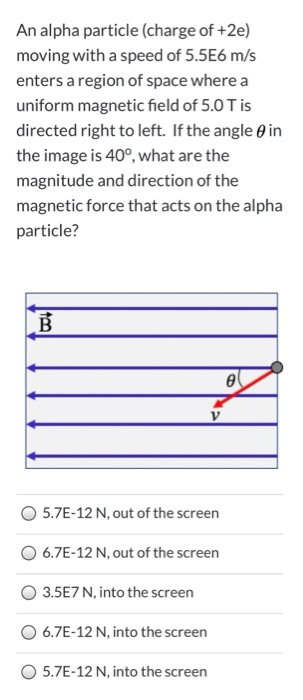An alpha particle (charge of +2e) moving with a speed of 5.5E6 m/s enters a region of space where a uniform magnetic field of 5.0 T is directed right to left. If the angle in the image is 40°, what are the magnitude and direction of the magnetic force that acts on the alpha particle? B e V 5.7E-12 N, out of the screen 6.7E-12 N, out of the screen 3.5E7 N, into the screen 6.7E-12 N, into the screen 5.7E-12 N, into the screen

Force on the charge particle is given as

F= q( v×B)= qvB *sin( theta)

So, F = 2*1.6*10^-19*5.5 *10^6* 5 *sin(40°)

F= 56.56*10^-13

Or F=5.7 *10 ^-12 N.

Putting your right hand fingers along v and curl it towards the B, we get force Direction, so force is in to the screen.

So last option is correct.

#### Earn Coin

Coins can be redeemed for fabulous gifts.

Similar Homework Help Questions
• ### An alpha particle of charge +2e and mass 6.64 × 10-27 kg is moving in the...

An alpha particle of charge +2e and mass 6.64 × 10-27 kg is moving in the +x direction at 1.03×106 m/s. It enters a region where the electric field is 2835 N/C in the -x direction. How far will the alpha particle travel before it comes to a stop? How much time will elapse from when the alpha particle enters the electric field to when it stops?

• ### An alpha particle of charge +2e and mass 6.64 × 10-27 kg is moving in the...

An alpha particle of charge +2e and mass 6.64 × 10-27 kg is moving in the +x direction at 1.84×106 m/s. It enters a region where the electric field is 1779 N/C in the -x direction. How far will the alpha particle travel before it comes to a stop? Tries 0/5 How much time will elapse from when the alpha particle enters the electric field to when it stops?

• ### A positive charge is moving with velocity v through a region of space where a uniform...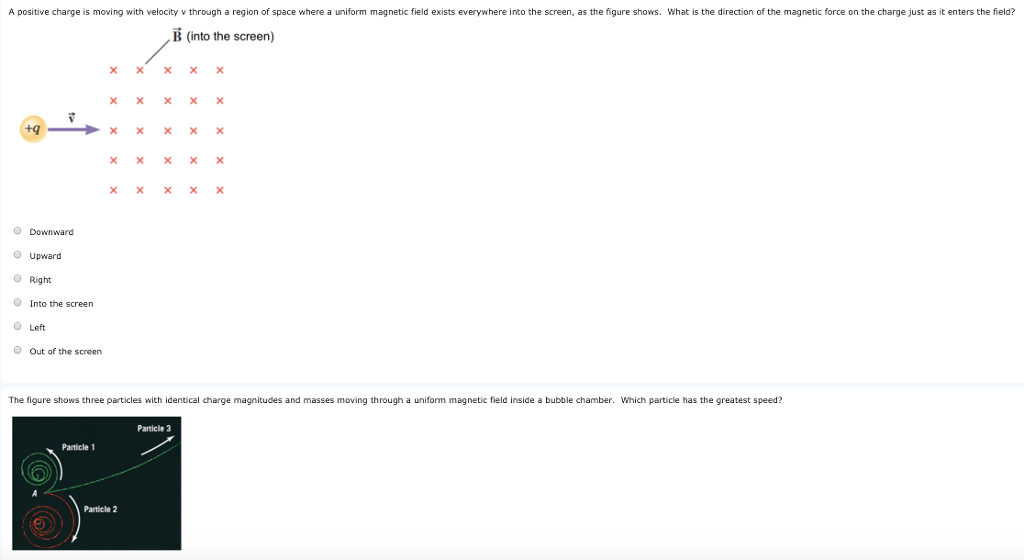A positive charge is moving with velocity v through a region of space where a uniform magnetic field exists everywhere into the screen, as the figure shows. What is the direction of the magnetic force on the charge just as it enters the field? B (into the screen) ㄨㄨㄨㄨㄨ ㄨㄨㄨㄨㄨ Downward Upward O Right O Into the screen Left O Out of the screen The figure shows three particles with identical charge magnitudes and masses moving through a uniform magnetic...

• ### An alpha particle of charge +2e and mass 6.64 x 1027 kg is moving in the...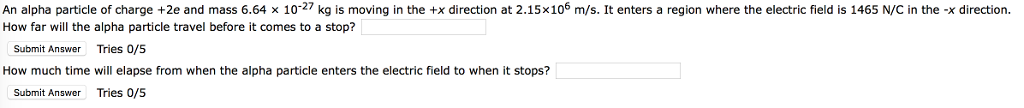An alpha particle of charge +2e and mass 6.64 x 1027 kg is moving in the +x direction at 2.15x106 m/s. It enters a region where the electric field is 1465 N/C in the -x direction. How far will the alpha particle travel before it comes to a stop? Submit Answer Tries 0/5 How much time will elapse from when the alpha particle enters the electric field to when it stops? Submit Answer Tries 0/5

• ### An alpha particle, which contains two protons and two neutrons (i.e., charge of +2e and mass...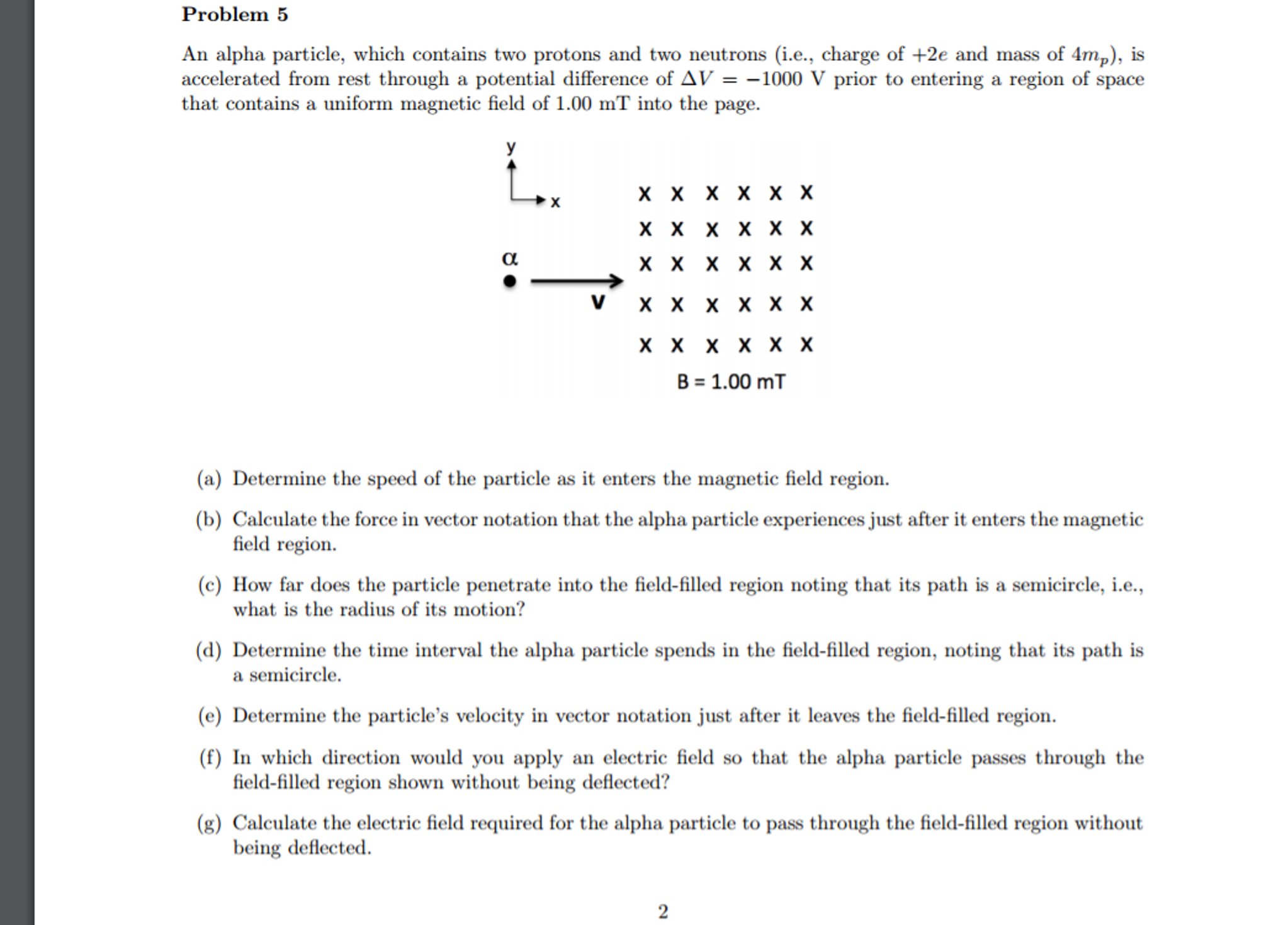An alpha particle, which contains two protons and two neutrons (i.e., charge of +2e and mass of 4m_p), is accelerated from rest through a potential difference of Delta V = -1000 V prior to entering a region of space that contains a uniform magnetic field of 1.00 mT into the page. Determine the speed of the particle as it enters the magnetic field region. Calculate the force in vector notation that the alpha particle experiences just after it enters the...

• ### An alpha-particle has a charge of +2e and a mass of 6.64 times 10^-27 kg. It...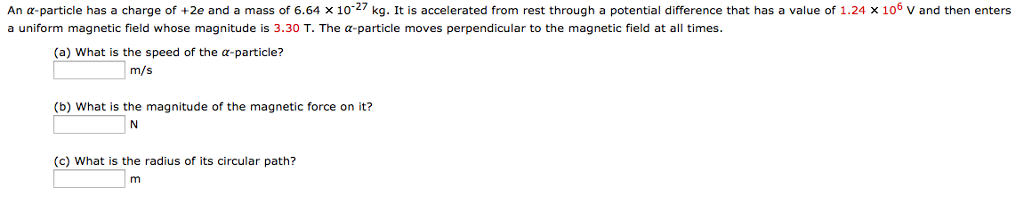An alpha-particle has a charge of +2e and a mass of 6.64 times 10^-27 kg. It is accelerated from rest through a potential difference that has a value of 1.24 times 10^6 v and then enters a uniform magnetic field whose magnitude is 3.30 T. The alpha-particle moves perpendicular to the magnetic field at all times. (a) What is the speed of the alpha-particle? m/s (b) What is the magnitude of the magnetic force on it? N (c) What is...

• ### An alpha-particle has a charge of +2e and a mass of 6.64 * 10^-27 kg. It...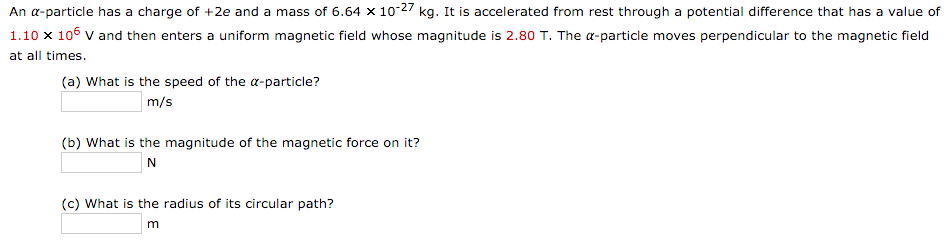An alpha-particle has a charge of +2e and a mass of 6.64 * 10^-27 kg. It is accelerated from rest through a potential difference that has a value of 1.10 * 10^6 V and then enters a uniform magnetic field whose magnitude is 2.80 T. The alpha-particle moves perpendicular to the magnetic field at all times. What is the speed of the alpha-particle What is the magnitude of the magnetic force on it What is the radius of its circular...

• ### A particle of charge q and mass m moves in a region of space where there...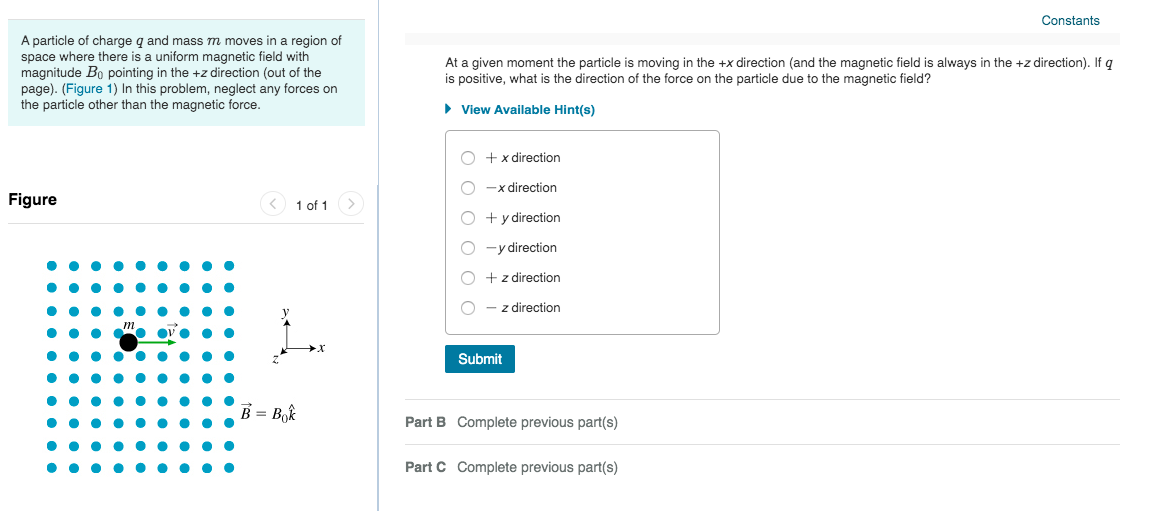A particle of charge q and mass m moves in a region of space where there is a uniform magnetic field with magnitude Bo pointing in the +z direction (out of the page) (Figure 1) In this problem, neglect any forces on the particle other than the magnetic force. At a given moment the particle is moving in the +x direction (and the magnetic field is always in the +z direction). If q is positive, what is the direction of the...

• ### A positive charge is moving with velocity v through a region of space where a uniform...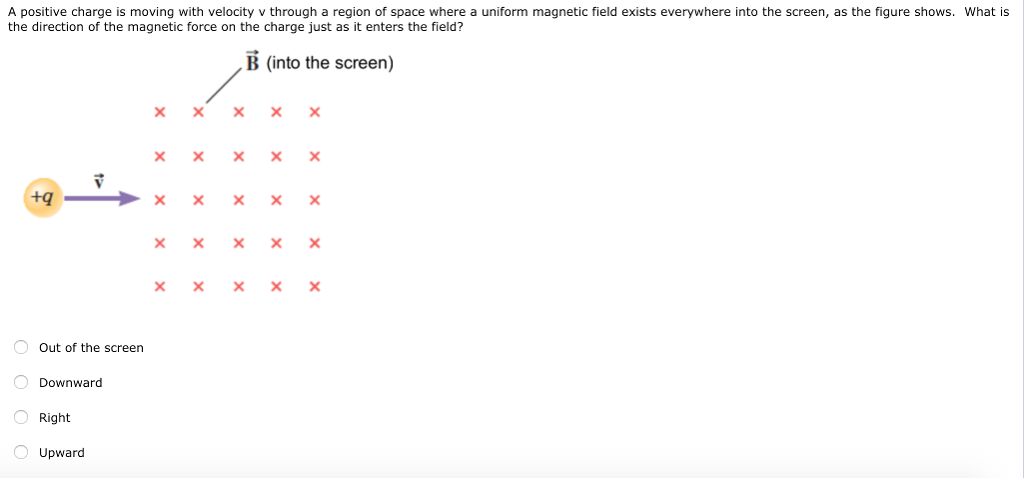A positive charge is moving with velocity v through a region of space where a uniform magnetic field exists everywhere into the screen, as the figure shows. What is the direction of the magnetic force on the charge just as it enters the field? B (into the screen) ㄨㄨㄨㄨㄨ ㄨㄨㄨㄨㄨ ㄨㄨㄨㄨㄨ Out of the screen Downward Right Upward

• ### A proton (charge le, mass mp) and an alpha particle (charge 2e, mass 4mp) in a...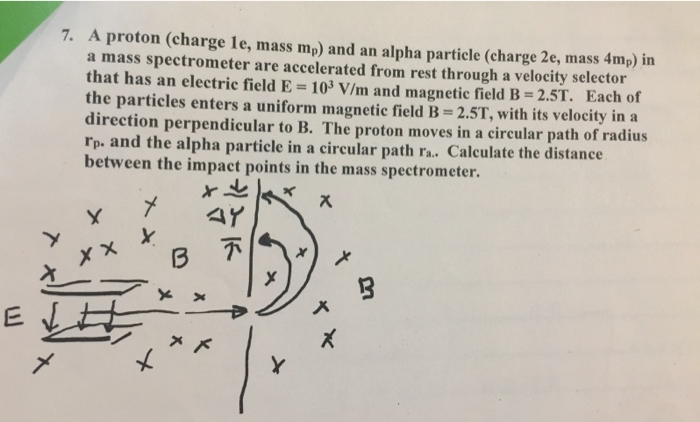A proton (charge le, mass mp) and an alpha particle (charge 2e, mass 4mp) in a mass spectrometer are accelerated from rest through a velocity selector that has an electric field E 103 V/m and magnetic field B- 2.5T. Each of the particles enters a uniform magnetic field B-2.5T, with its velocity in a direction perpendicular to B. The proton moves in a circular path of radius rp. and the alpha particle in a circular path ra. Calculate the distance...